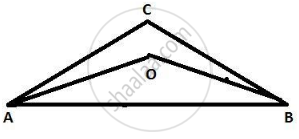Advertisement Remove all ads

# In δ Abc, Bisectors of ∠ a and ∠ B Intersect at Point O. If ∠ C = 70° . Find Measure of ∠ Aob. - Geometry

Sum

In Δ ABC, bisectors of ∠ A and ∠ B intersect at point O. If ∠ C = 70° . Find measure of ∠ AOB.

Advertisement Remove all ads

#### SolutionSince we have given that

ABC is a triangle.

Bisectors of ∠A and ∠B intersect at point O.

So, a measure of ∠C = 70°.

So, ∠AOB would be

The measure of ∠AOB is given by

90^circ + (angle C)/2

= 90^circ + 70/2

= 90^circ + 35^circ

= 125^circ

Hence, the measure of ∠AOB = 125°.

Is there an error in this question or solution?
Advertisement Remove all ads

#### APPEARS IN

Balbharati Mathematics 2 Geometry 9th Standard Maharashtra State Board
Chapter 3 Triangles
Practice Set 3.1 | Q 7 | Page 28
Advertisement Remove all ads
Advertisement Remove all ads
Share
Notifications

View all notifications

Forgot password?